Skip to content
Related Articles
R – Bar Charts
• Last Updated : 21 Apr, 2020

A bar chart is a pictorial representation of data that presents categorical data with rectangular bars with heights or lengths proportional to the values that they represent. In other words, it is the pictorial representation of dataset. These data sets contain the numerical values of variables that represent the length or height.

R uses the function barplot() to create bar charts. Here, both vertical and Horizontal bars can be drawn.

Syntax:

`barplot(H, xlab, ylab, main, names.arg, col)`

Parameters:

• H: This parameter is a vector or matrix containing numeric values which are used in bar chart.
• xlab: This parameter is the label for x axis in bar chart.
• ylab: This parameter is the label for y axis in bar chart.
• main: This parameter is the title of the bar chart.
• names.arg: This parameter is a vector of names appearing under each bar in bar chart.
• col: This parameter is used to give colors to the bars in the graph.

### Creating a Simple Bar Chart

Approach: In order to create a Bar Chart:

1. A vector (H <- c(Values…)) is taken which contain numeral values to be used.
2. This vector H is plot using barplot().

Example:

 `# Create the data for the chart``A <``-` `c(``17``, ``32``, ``8``, ``53``, ``1``)`` ` `# Plot the bar chart ``barplot(A, xlab ``=` `"X-axis"``, ylab ``=` `"Y-axis"``, main ``=``"Bar-Chart"``)`

Output: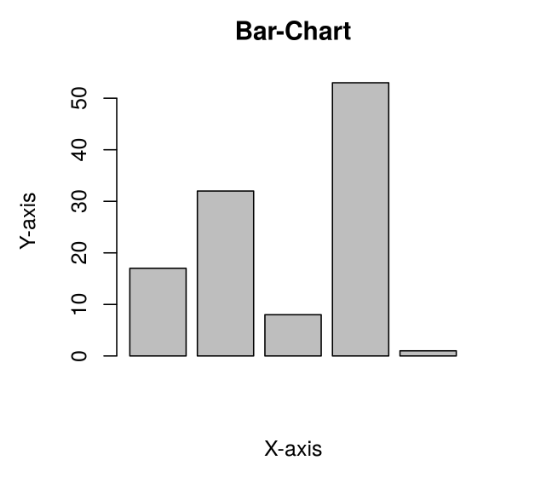### Creating a Horizontal Bar Chart

Approach: To create a horizontal bar chart:

1. Take all parameters which are required to make simple bar chart.
2. Now to make it horizontal new parameter is added.
`barplot(A, horiz=TRUE )`

Example: Creating a horizontal bar chart

 `# Create the data for the chart``A <``-` `c(``17``, ``32``, ``8``, ``53``, ``1``)``  ` `# Plot the bar chart ``barplot(A, horiz ``=` `TRUE, xlab ``=` `"X-axis"``,``        ``ylab ``=` `"Y-axis"``, main ``=``"Bar-Chart"``)`

Output: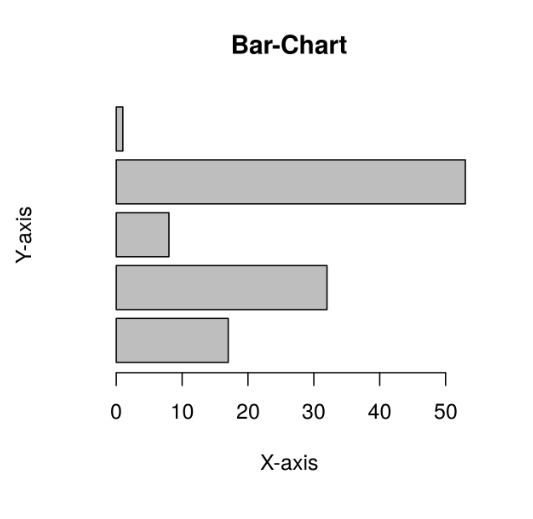### Adding Label, Title and Color in the BarChart

Label, title and colors are some properties in the bar chart which can be added to the bar by adding and passing an argument.
Approach:

1. To add the title in bar chart.
`barplot( A, main = title_name )`
2. X-axis and Y-axis can be labeled in bar chart. To add the label in bar chart.
`barplot( A, xlab= x_label_name, ylab= y_label_name)`
3. To add the color in bar chart.
`barplot( A, col=color_name)`

Example :

 `# Create the data for the chart``A <``-` `c(``17``, ``2``, ``8``, ``13``, ``1``, ``22``)``B <``-` `c(``"Jan"``, ``"feb"``, ``"Mar"``, ``"Apr"``, ``"May"``, ``"Jun"``)`` ` `# Plot the bar chart ``barplot(A, names.arg ``=` `B, xlab ``=``"Month"``, ``        ``ylab ``=``"Articles"``, col ``=``"green"``, ``        ``main ``=``"GeeksforGeeks-Article chart"``)`

Output: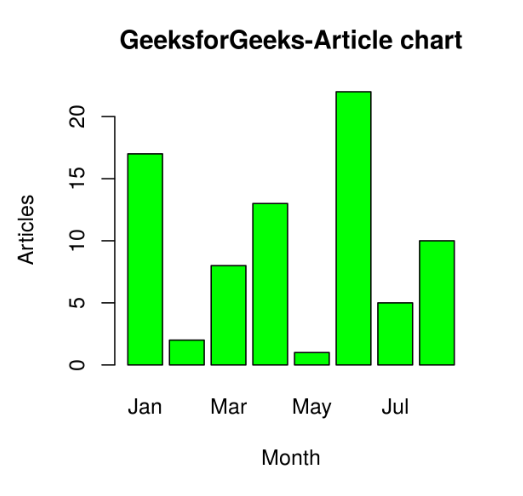### Creating Stacked and Grouped Bar Chart

The bar chart can be represented in two form group of bars and stacked.
Approach:

1. Take a vector value and make it matrix M which to be grouped or stacked. Making of matrix can be done by.
`M <- matrix(c(values...), nrow = no_of_rows, ncol = no_of_column, byrow = TRUE)`
2. To display the bar explicitly we can use the beside parameter.
`barplot( beside=TRUE )`

Example 1:

 `colors ``=` `c(``"green"``, ``"orange"``, ``"brown"``)``months <``-` `c(``"Mar"``, ``"Apr"``, ``"May"``, ``"Jun"``, ``"Jul"``)``regions <``-` `c(``"East"``, ``"West"``, ``"North"``)`` ` `# Create the matrix of the values.``Values <``-` `matrix(c(``2``, ``9``, ``3``, ``11``, ``9``, ``4``, ``8``, ``7``, ``3``, ``12``, ``5``, ``2``, ``8``, ``10``, ``11``), ``                 ``nrow ``=` `3``, ncol ``=` `5``, byrow ``=` `TRUE)`` ` `# Create the bar chart``barplot(Values, main ``=` `"Total Revenue"``, names.arg ``=` `months, ``                          ``xlab ``=` `"Month"``, ylab ``=` `"Revenue"``, ``                          ``col ``=` `colors, beside ``=` `TRUE)`` ` `# Add the legend to the chart``legend(``"topleft"``, regions, cex ``=` `0.7``, fill ``=` `colors)`

Output: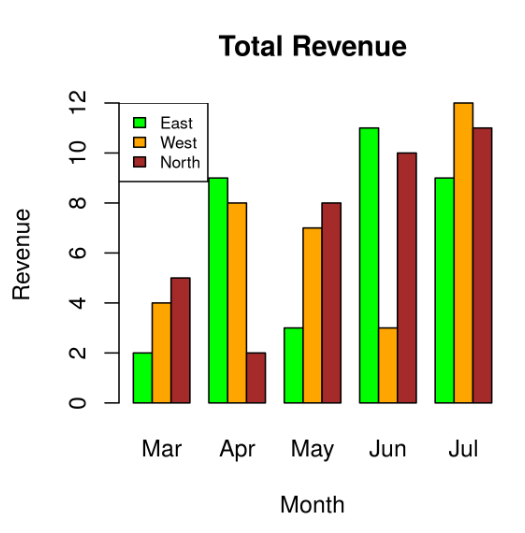Example 2:

 `colors ``=` `c(``"green"``, ``"orange"``, ``"brown"``)``months <``-` `c(``"Mar"``, ``"Apr"``, ``"May"``, ``"Jun"``, ``"Jul"``)``regions <``-` `c(``"East"``, ``"West"``, ``"North"``)``  ` `# Create the matrix of the values.``Values <``-` `matrix(c(``2``, ``9``, ``3``, ``11``, ``9``, ``4``, ``8``, ``7``, ``3``, ``12``, ``5``, ``2``, ``8``, ``10``, ``11``),``                   ``nrow ``=` `3``, ncol ``=` `5``, byrow ``=` `TRUE)``  ` `# Create the bar chart``barplot(Values, main ``=` `"Total Revenue"``, names.arg ``=` `months, ``        ``xlab ``=` `"Month"``, ylab ``=` `"Revenue"``, col ``=` `colors)``  ` `# Add the legend to the chart``legend(``"topleft"``, regions, cex ``=` `0.7``, fill ``=` `colors)`

Output: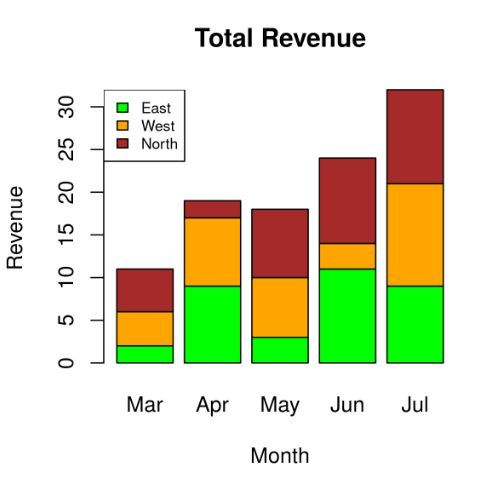My Personal Notes arrow_drop_up